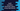# How to transform swift dictionary values using mapValues()# How to transform swift dictionary values using mapValues()

Swift dictionary provides map method to iterate over its elements and convert it to a different key-value pairs. This method returns one array of key-value items. Other than that method, we have one more method called mapValues that is used to modify only the values, not keys.

Unlike map(), this method returns one new dictionary. Because, we are not changing the keys, so it will just modify the values and return that new dictionary.

## Definition of mapValues() :

mapValues() is defined as below :

``func mapValues<T>(_ transform: (Value) throws -> T) rethrows -> Dictionary<Key, T>``

It takes only one parameter : transform. This is a closure that takes each value of the dictionary, modifies it (or not) and returns that new value. Easy - just use one closure to change the values and it will return you one new dictionary.

If you want to change only the values of a dictionary not both keys and values, then this method makes more sense.

### Example of mapValues() :

Let’s take a look at the below example :

``````var my_dict = ["key_1" : 1, "key_2" : 2, "key_3": 3]

print(my_dict.mapValues{\$0 * 3})``````

Here, my_dict is a dictionary given. Using mapValues, we are multiplying the values of each element by 3. It will print :

``["key_3": 9, "key_2": 6, "key_1": 3]``

It’s complexity is O(n), where n is the length of the dictionary.